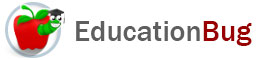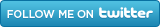PUBLIC SCHOOLS PRIVATE SCHOOLS SCHOOL DISTRICTS COLLEGES PUBLIC LIBRARIES JOBS BLOG RESOURCES
Career Education
Education Choices
Education Costs
Education History and General Information
Education Issues
Education Resources
Family Involvement in Education
Students w Special Needs
Subject Information and Homework Help
Teaching and Learning
Testing
By Types of Schools
By Specific Audiences
GeneralRounding Numbers

Solving math problems may require rounding numbers for a variety of reasons. This article will define a round number, give ways of rounding numbers, and explain rounding numbers by digit.

Have you ever heard anyone ask for a â€œballpark figureâ€? Itâ€™s one way of saying that an approximation, rather than an exact amount, will be suitable for whatever the particular purpose is. Approximations can be achieved in several ways. One is by doing a rough measurement. Another is by rounding numbers. This article explores this, and other reasons for rounding numbers.

A Round Number

Round numbers means two different things. First it refers to numbers that end in zeroes without any alteration, such as the calendar year 2000. In addition, it refers to numbers that have undergone the process of rounding, which may or may not result in the number ending in a zero. It is the second type of round number that we are concerned with in this article.

As hinted at earlier, one way of rounding numbers is to do a rough estimate while measuring. If, for example, you know that your arm length is about a yard, and-perhaps not having a measuring tape-you use your arm to measure a distance and ignore the little bit extra at the end, announcing that the distance is about nine feet, thatâ€™s one way of rounding a number.

Rounding can also occur when a precise number is known and the person using the number chooses not to work at that level of precision. One reason for doing this is that such a precise answer is not needed. Another is that other numbers being used in a formula along with the precise number are not as precise. A third is that a number with less digits is easier to use.

Ways of Rounding Numbers

There are two ways of rounding that most people may use at some point: rounding to the nearest, specified place or rounding to a determined number of significant figures (also called significant digits). Because rounding to significant figures is a more specialized, scientific approach, we will concentrate on the more general approach.

Rounding Numbers by Digit

When rounding is first taught in school, it is common to have problems that ask the student to â€œround to the nearest tenthâ€ or â€œround to a whole number.â€ The method for doing this has some variations, but it basically goes like this:

1) Identify the digit that will be the final digit in the rounded number. If youâ€™re rounding to tenths, then you will end up with only one number to the right of the decimal point. If youâ€™re rounding to a whole number, there will be no decimal places.

2) look at the digit immediately to the right of the digit that will become the final digit.

a) if this digit is greater than or equal to five, raise the final digit by one and eliminate all digits to the right, including the one you just considered. For example,

349.26 rounded to the nearest tenth would be 349.3

What happens if the digit youâ€™re adding one to is a 9? Then you add one and do any necessary carrying:

349.96 rounded to the nearest tenth would be 350.0

This seems odd, because four digits have changed: one has been eliminated (the hundredths place); two have changed to zero (the ones and tenths place) and one has changed from 4 to 5 (the tens place). But it follow the logic of the operation.

Notice that the tenths place is preserved, even though it is zero. Also note that different systems may elect to have different approaches taken if the digit to the right of the final digit is 5.

b) if this digit is less than five, leave the final digit as it is and eliminate all digits to the right, including the one you just considered. For example,

349.233389999945878999 rounded to the nearest tenth would be 349.2

Notice that it doesnâ€™t matter how many digits are eliminated in this process or whether any of them after the digit you are considering when you round are greater than or equal to five. This is true even if the numbers being eliminated are to the left of the decimal point:

12,499,999,999 people rounded to the nearest billion is 12,000,000,000 even though that means that 499,999,999 people are dropped from the reckoning by that change. One more person, and the rounded number would have been 13,000,000,000. This suggests one reason that itâ€™s important to consider carefully what is being gained and what may be lost when one is rounding.

Related Articles
Subtraction